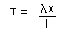## Elastic Strings

Modulus and Natural Length

Elastic strings are strings which are not a fixed length (they can be stretched). Some strings are more stretchy than others and the modulus (or modulus of elasticity) of a string is a measure of how stretchy it is. The modulus is measured in newtons.

The length of an elastic string which does not have any forces acting upon it is known as the natural length of the string. If a string has been stretched, then the extension is how much longer the string is as a result of being stretched. Note that the extension = length of the string - natural length.

Hooke's Law

Hooke's law states that the tension in an elastic string (or spring), T, is found using the following formula:, where l is the modulus of elasticity of the string, x is the extension of the string and l is the natural length of the string.

Example

A string with modulus (of elasticity) 10 N has a natural length of 2m. What is the tension in the string when its length is 5m?

T = 10 × 3 = 15
2

So the tension in the string is 15N.

Potential Energy Stored in String

When an elastic string is extended it has elastic potential energy.

• Elastic potential energy stored in string = lx2/2l

In problems involving strings (and springs), if the only external force doing work is gravity then energy is conserved. Hence elastic potential energy + gravitational potential energy + kinetic energy = constant.

Springs

What has been said about strings also applies to springs. However, springs can be compressed as well as stretched. If a spring is compressed, then Hooke's Law still applies but T represents the "thrust" rather than the tension (basically the only difference is that thrust acts in the opposite direction to tension).